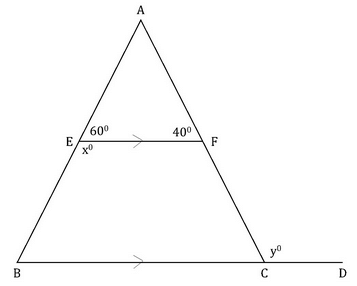In the given diagram, line segment EF and BC ...In the given diagram, line segment EF and BC are parallel to each other. Find the value of (x+y).
• a)
100o
• b)
120o
• c)
140o
• d)
240o
• e)
360o
Correct answer is 'E'. Can you explain this answer?

### Related TestSamraggi Kumar May 27, 2018
Related In the given diagram, line segment EF and BC are parallel to each other. Find the value of (x+y).a)100ob)120oc)140od)240oe)360oCorrect answer is 'E'. Can you explain this answer?
X=180-60=120 angle e=angle b =60 angle a = 180-(60+40)=80 angle a+ angle b=y y =80+60=140 x+y=140+120=260

 1 Crore+ students have signed up on EduRev. Have you?Rahul Prajapati Aug 14, 2019
Answer is misprinted, correct answer is 260 degreePreitee Ranjan Pradhan Jun 11, 2020
Related In the given diagram, line segment EF and BC are parallel to each other. Find the value of (x+y).a)100ob)120oc)140od)240oe)360oCorrect answer is 'E'. Can you explain this answer?
260Abdurrahman Joomye Aug 17, 2022
Correct answer is 260
x = 180 - 60 = 120
y = EFC = 180 - 40 = 140
x + y = 120 + 140 = 260

Question Description
In the given diagram, line segment EF and BC are parallel to each other. Find the value of (x+y).a)100ob)120oc)140od)240oe)360oCorrect answer is 'E'. Can you explain this answer? for GMAT 2022 is part of GMAT preparation. The Question and answers have been prepared according to the GMAT exam syllabus. Information about In the given diagram, line segment EF and BC are parallel to each other. Find the value of (x+y).a)100ob)120oc)140od)240oe)360oCorrect answer is 'E'. Can you explain this answer? covers all topics & solutions for GMAT 2022 Exam. Find important definitions, questions, meanings, examples, exercises and tests below for In the given diagram, line segment EF and BC are parallel to each other. Find the value of (x+y).a)100ob)120oc)140od)240oe)360oCorrect answer is 'E'. Can you explain this answer?.
Solutions for In the given diagram, line segment EF and BC are parallel to each other. Find the value of (x+y).a)100ob)120oc)140od)240oe)360oCorrect answer is 'E'. Can you explain this answer? in English & in Hindi are available as part of our courses for GMAT. Download more important topics, notes, lectures and mock test series for GMAT Exam by signing up for free.
Here you can find the meaning of In the given diagram, line segment EF and BC are parallel to each other. Find the value of (x+y).a)100ob)120oc)140od)240oe)360oCorrect answer is 'E'. Can you explain this answer? defined & explained in the simplest way possible. Besides giving the explanation of In the given diagram, line segment EF and BC are parallel to each other. Find the value of (x+y).a)100ob)120oc)140od)240oe)360oCorrect answer is 'E'. Can you explain this answer?, a detailed solution for In the given diagram, line segment EF and BC are parallel to each other. Find the value of (x+y).a)100ob)120oc)140od)240oe)360oCorrect answer is 'E'. Can you explain this answer? has been provided alongside types of In the given diagram, line segment EF and BC are parallel to each other. Find the value of (x+y).a)100ob)120oc)140od)240oe)360oCorrect answer is 'E'. Can you explain this answer? theory, EduRev gives you an ample number of questions to practice In the given diagram, line segment EF and BC are parallel to each other. Find the value of (x+y).a)100ob)120oc)140od)240oe)360oCorrect answer is 'E'. Can you explain this answer? tests, examples and also practice GMAT tests.